# NCERT Solutions for Class 12 Physics Chapter 1 Electric Charges and Fields

In this chapter, we provide NCERT Solutions for Class 12 Physics for English medium students, Which will very helpful for every student in their exams. Students can download the latest NCERT Solutions for Class 12 Physics pdf, free NCERT solutions for Class 12 Physics book pdf download. Now you will get step by step solution to each question. Class 12 physics is a very important subject for entrance exams like IIT JEE, CPMT, etc.

## NCERT Solutions for Class 12 Physics Chapter 1 Electric Charges And Fields

NCERT Exercises

Question 1.
What is the force between two small charged spheres having charges of 2 × 10-7 C and 3 × 10-7 C placed 30 cm apart in air?

Solution: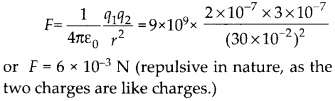Question 2.
The electrostatic force on a small sphere of charge 0.4 μC due to another small sphere of charge -0.8 μC in air is 0.2 N.
(a)What is the distance between the two spheres?
(b)What is the force on the second sphere due to the first?
Solution:Question 3.
Check that the ratio$\frac { Ke^{ 2 } }{ Gm_{ e }m_{ p } }$ is dimensionless. Look up a table of Physical Constants and determine the value of this ratio. What does the ratio signify?
Solution:
The ratio of electrostatic force to the gravitational force between an electron and a proton separated by a distance r from each other is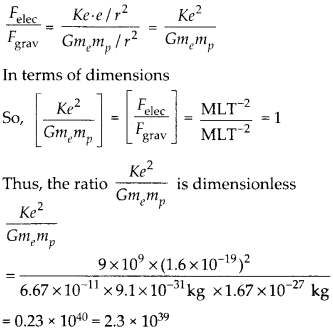This ratio signifies that electrostatic forces are 1039 times stronger than gravitational forces.

Question 4.
(a) Explain the meaning of the statement ‘electric charge of a body is quantised’.
(b) Why can one ignore quantisation of electric charge when dealing with macroscopic i.e., large scale charges?
Solution:
(a) The net charge possessed by a body is an integral multiple of charge of an electron i.e. q = ± ne, where n = 0,1,2,3,… is the number of electrons lost gained by the body and e = 1.6 × 10-19 C is charge of an electron. This is called law of quantization of charge.
(b) At macroscopic level, charges are enormously large as compared to the charge of an electron, e = 1.6 × 10-19 C. Even a charge of 1 nC contains nearly 1013 electronic charges. So, at this large scale, charge can have a continuous value rather than discrete integral multiple of e, and hence, the quantization of electric charge can be ignored.

Question 5.
When a glass rod is rubbed with a silk cloth, charges appears on both. A similar phenomenon is observed with many other pairs of bodies. Explain how this observation is consistent with the law of conservation of charge.
Solution:
When a glass rod is rubbed with a silk cloth, electrons from the glass rod are transferred to the piece of silk cloth. Due to this, the glass rod acquires positive (+) charge whereas the silk cloth acquires negative (-) charge. Before rubbing, both the glass rod and silk cloth are neutral and after rubbing the net charge on both of them is also equal to zero. Such similar phenomenon is observed with many other pairs of bodies. Thus, in an isolated system of bodies, charge is neither created nor destroyed, it is simply transferred from one body to the other. So, it is consistent with the law of conservation of charge.

Question 6.
Four point charges qA = + 2 μC, qB = -5μC, qc = + 2 μC and qD = -5 μC are located at the corners of a square ABCD of side 10 cm. What is the force on a charge of 1 μC placed at the centre of the square?
Solution:Forces of repulsion on 1 μC charge at O due to 2 μC charge, at A and C are equal and opposite. Therefore, they cancel. Similarly, forces of attraction on 1 μC charge at O, due to -5 μC charges at B and at D are also equal and opposite. Therefore, these also cancel. Hence, the net force on the charge of 1 μC at O is zero.

Question 7.
(a) An electrostatic field line is a continuous curve. That is, a field line cannot have sudden breaks. Why not?
(b)Explain why two field lines never cross each other at any point?
Solution:A field line cannot have sudden breaks because the moving test charge never jumps from one position to the other.
(b)Two field lines never cross each other at any point/because if they do so, we will obtain two tangents pointing in two different directions of electric field at a point, which is not possible.

Question 8.
Two point charges qA = 3 μC and qB = -3 μC are located 20 cm apart in vacuum.
(a)What is the electric field at the midpoint O of the line AS joining the two charges?
(b)If a negative test charge of magnitude 1.5 × 10-9 C is placed at this point, what is the force experienced by the test charge?
Solution:
(a) Electric fields at O due to the charges at A and B areIn direction opposite to that of E i.e. along OA.

Question 9.
A system has two charges qA = 2.5 × 10-7 C and qB = -2.5 × 10-7 C located at point A (0,0, -15 cm) and B (0,0, +15 cm), respectively. What are the total charge and electric dipole moment of the system?
Solution: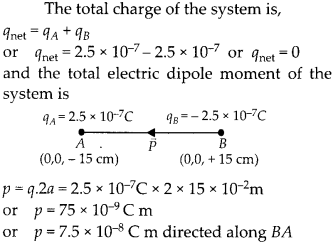Question 10.
An electric dipole with dipole moment 4 × 10-9 C m is aligned at 30° with the direction of a uniform electric field of magnitude 5 × 104 N C-1. Calculate the magnitude of the torque acting on the dipole.

Solution: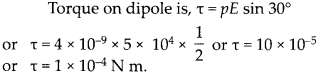Question 11.
A Polythene piece rubbed with wool is found to have a negative charge of 3 × 10-7 C.
(a) Estimate the number of electrons transferred (from which to which?)
(b) Is there a transfer of mass from wool to polythene?
Solution:
(a) q = ne or n =$\frac { q }{ e } =\frac { 3\times 10^{ -7 } }{ 1.6\times 10^{ -19 } }$
= 1.875 × 1012
so, 1.875 × 1012 electrons have transferred from wool to polythene, as polythene acquires negative charge.
(b) Yes, mass of 1.875 × 1012 electrons i.e., m = nme = 1.875 × 1012 × 9.1 × 10-3 1 kg = 1.71 × 10-18 kg has transferred from wool to polythene

Question 12.
(a) Two insulated charged copper spheres A and B have their centres separated by a distance of 50 cm. What is the mutual force of electrostatic repulsion if the charge on each is 6.5 × 10-7 C ? The radii of A and B are negligible compared to the distance of separation.
(b) What is the force of repulsion if each sphere is charged double the above amount, and the distance between them is halved?
Solution: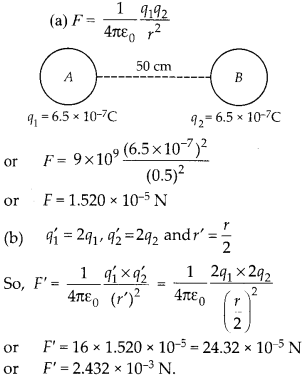Question 13.
Suppose the spheres A and B in previous question have identical sizes. A third sphere of the same size but uncharged is brought in contact with the first, then brought in contact with the second, and finally removed from both. What is the new force of repulsion between A and B?
Solution: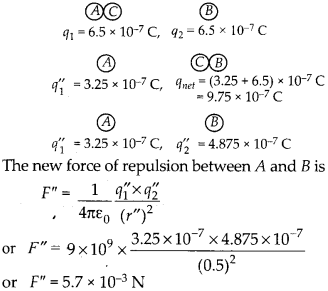Question 14.
Figure shows tracks of three charged particles in a uniform electrostatic field. Give the signs of the three charges. Which particle has the highest charge to mass ratio?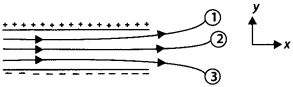Solution:
Particles 1 and 2 are negatively charged as they experience forces in direction opposite to that of electric field E, whereas particle 3 is positively charged as it experience force in the direction of electric field E.Particle-3 has the highest charge to mass ratio, as it shows maximum deflection in the electric field.

Question 15.
Consider a uniform electric field$\overrightarrow { E } =3\times 10^{ 3 }\hat { i } NC^{ -1 }$.
(a)What is the flux of this field through a square of 10 cm on a side whose plane is parallel to the yz plane?
(b)What is the flux through the same square if the normal to its plane makes a 60° angle with the x-axis?
Solution:Question 16.
What is the net flux of the uniform electric field in previous question through a cube of side 20 cm oriented so that its faces are parallel to the coordinate planes?
Solution:${ \phi }_{ net }=0,$ As the net electric flux with closed surface like cube in uniform electric
field is equal to zero, because the number of lines entering the cube is the same as the number of lines leaving the cube.

Question 17.
Careful measurement of the electric field at the surface of a black box indicates that the net outward flux though the surface of the box is 8.0 × 103 N m2 C-1.
(a)What is the net charge inside the box?
(b)If the net outward flux through the surface of the box were zero, could you conclude that there were no charges inside the box? Why or why not?
Solution: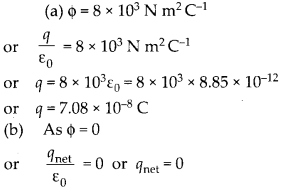So, the net charge enclosed by that closed surface is zero, although it may have some charges inside it.

Question 18.
A point charge +10 μC is at a distance of 5 cm directly above the centre of a square of side 10 cm, as shown in figure. What is the magnitude of the electric flux though the square?
Solution:
Let us assume that the given square be one face of the cube of edge 10 cm. As charge of +10 μC is at a distance of 5 cm above the centre of a square, so it is enclosed by the cube. Hence by Gauss’s theorem, electric flux linked with the cube isQuestion 19.
A point charge of 2.0 pC is at the centre of a cubic Gaussian surface 9.0 cm on edge. What is the net electric flux through the surface?
Solution: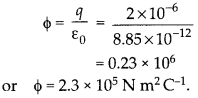Question 20.
A point charge causes an electric flux of -1.0 × 103 Nm2 C-1 to pass through a spherical Gaussian surface of 10.0 cm radius centred on the charge.
(a) If the radius of the Gaussian surface were doubled, how much flux would pass through the surface?
(b) What is the value of the point charge?
Solution:
(a) On increasing the radius of the Gaussian surface, charge enclosed by it remains the same and hence the electric flux linked with Gaussian surface also remains the same.Question 21.
A conducting sphere of radius 10 cm has an unknown charge. If the electric field 20 cm from the centre of the sphere is 1.5 × 103 N C-1 and points radially inward. What is the net charge on the sphere?

Solution: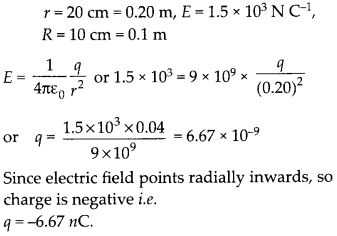Question 22.
A uniformly charged conducting sphere of 2.4 m diameter has a surface charge density of 80.0 μC m-2.
(a) Find the charge on the sphere.
(b) What is the total electric flux leaving the surface of the sphere?

Solution: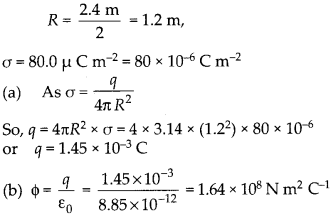Question 23.
An infinite line charge produces a field of 9 × 104NC-1 at a distance of 2 cm. Calculate the linear charge density.
Solution: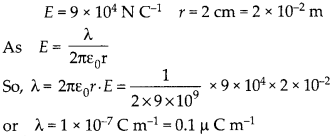Question 24.
Two large, thin metal plates are parallel and close to each other. On their inner faces, the plates have surface charge densities of opposite signs and of magnitudes 17.0 × 10-22 C m-2. What is Electric field in
(a) the outer region of the first plate.
(b) the outer region of the second plate, and
(c) between the plates?
Solution:
As both the plates have same surface charge density σ, so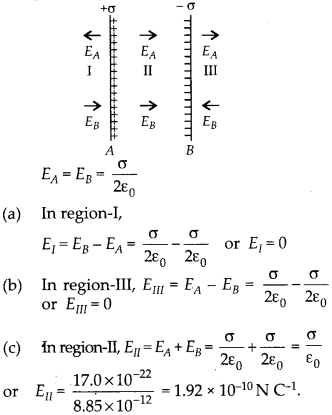Question 25.
An oil drop of 12 excess electrons is held stationary under a constant electric field of 2.55 × 104 N C-1 in Millikan’s oil drop experiment. The density of the oil is 1.26 g cm-3. Estimate the radius of the drop.
(g = 9.81 m s-2; e= 1.6 × 10-19 C)
Solution:
In equilibrium, force due to electric field on the drop balances the weight mg of dropQuestion 26.
Which among the curves shown in Figure cannot possibly represent electrostatic field lines?

Solution:
(a) It is wrong, because electric field lines must be normal to the surface of conductor outside it.
(b) It is wrong because field lines cannot start or originate from negative charge, and also cannot end or submerge into positive charge.
(c) It is correct
(d) It is wrong because electric field lines never intersect each other.
(e) It is wrong because electric field lines cannot form closed loops.

Question 27.
In a certain region of space, electric field is along the z-direction throughout. The magnitude of electric field is, however, not constant but increases uniformly along the positive z-direction, at the rate of 105 N C-1 per metre. What are the force and torque experienced by a system having a total dipole moment equal to 10-7 C m in the negative z-direction?
Solution:
As electric field increases in positive z-direction from A to B, Sodirected in direction of FB i.e., from B to A or along -z direction.
As the two forces on charges of electric dipole are collinear and opposite, so net torque on it is equal to zero.

Question 28.
(a) A conductor A with a cavity is shown in figure (a) is given a charge Q. Show that the entire charge must appear on the outer surface of the conductor.
(b) Another conductor B with charge q is inserted into the cavity keeping B insulated from A. Show that the total charge on the outside surface of A is Q + q. (figure (b))
(c) A sensitive instrument is to be shielded from the strong electrostatic fields in its environment. Suggest a possible way.
Solution:
(a) Let us consider a closed surface inside the conductor enclosing the cavity. As electric field inside the conductor is zero,This shows that there cannot be any charge on the inner surface of conductor at the cavity, so any charge Q given to the conductor will appear only on its outer surface.
(b) Let us consider that the charge -qI is induced on the inner surface of conductor A at the cavity, when conductor B of charge q is kept in its cavity. Due to this charge of Q + qt is induced on the outer surface of conductor. Let us construct a closed Gaussian surface S inside the conductor A enclosing its cavity. As, electric field inside conductor is zero, soi.e., equal and opposite charge, -q is induced on inner surface of conductor A at the cavity,

when conductor B of charge, + q is kept is its cavity. Hence, the charge on outer surface of conductor is Q + q1 = Q + q
(c) As we found that electric field inside the cavity of conductor is zero, even on charging the conductor, so the sensitive instrument can be shielded from the strong electrostatic fields in its environment, by covering it with a metallic cover.

Question 29.
A hollow charged conductor has a tiny hole cut into its surface. Show that the electric field in the hole is$\left( \frac { \sigma }{ 2\varepsilon _{ 0 } } \hat { n } \right)$ .where$\hat { n }$ is the unit vector in the outward normal direction, and o is the surface charge density near the hole.
Solution:
Inside a charged conductor, the electric field is zero.But a uniformly charged flat surface provideIf we consider a small flat part on the surface of charged conductor, it certainly provides anNow if a hole is made in charged conductor, the fifeld due to small flat part is absent but the field due to rest of charged conductor is present i.e., equal to$\frac { \sigma }{ 2\varepsilon _{ 0 } } \hat { n }$.

Question 30.
Obtain the formula for the electric field due to a long thin wire of uniform linear charge density X without using Gauss’s law.
Solution:
Consider a point P, a unit away from the long charged wire. Electric field due to element dy,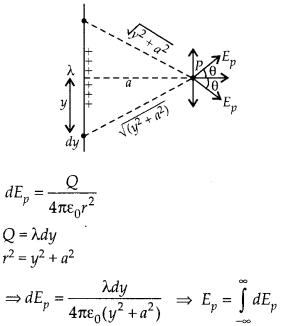vertical components cancel out and horizontal components are added due to symmetryQuestion 31.
It is now believed that protons and neutrons (which constitute nuclei of ordinary matter) are themselves built out of more elementary units called quarks. A proton and a neutron consist of three quarks each. Two types of quarks, the so called ‘up’ quark (denoted by u) of charge + 2/3 e, and the ‘down’ quark (denoted by d) of charge (-1/3)e, together with electrons build up ordinary matter. [Quarks of other types have also been found which give rise to different unusual varieties of matter]. Suggest a possible quark composition of a proton and neutron.
Solution: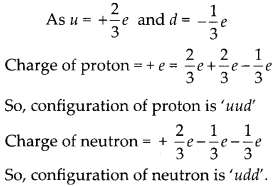Question 32.
(a) Consider an arbitrary electrostatic field configuration. A small test charge is placed at a null point (i.e., where E = o) of the configuration. Show that the equilibrium of the test charge is necessarily unstable, (b) Verify this result for the simple configuration of two charges of the same magnitude and sign placed at certain distance apart.
Solution:
(a) Let us assume that a radial electric field is present with origin as centre. The E is 0 at origin and a small test charge ‘q0’ is placed at origin. Force on test charge at origin is zero.$\overrightarrow { F } =q\overrightarrow { E } =0$ But as the test charge is displaced a little, the electrostatic force will drift it further away so charge is in unstable equilibrium.

(b) A test charge placed at mid point of equal charges is in stable equilibrium, for lateral displacement. Initially at mid point position of test charge, two repulsion forces on test charge are equal and opposite.

But on displacing q0 close to one of the charge, one of the forces become stronger and pushes the charge towards another.FA > FB so q0 moves towards B.

Question 33.
A particle of mass m and charge (-q) enters the region between the two charged plates initially moving along x-axis with speed vx.The length of plate is L and an uniform electric field £ is maintained between the plates. Show that the vertical deflection of the particle at the far edge of the plate is qEL2/$2m{ v }_{ x }^{ 2 }$.
Solution:
Let the point at which the charged particle enters the electric field, be origin O (0, 0), then after travelling a horizontal displacement L, it gets deflected by displacement y in vertical direction as it comes out of electric field. So, co-ordinates of its initial position are x1 = 0 and y1 = 0 and final position on coming out of electric field are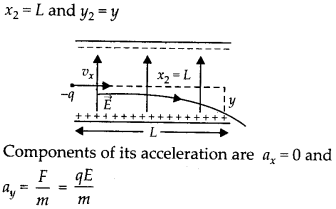and of initial velocity are ux = vx and uy = 0 so, by 2nd equation of motion in horizontal direction,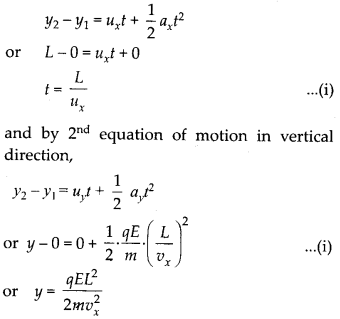This gives the vertical deflection of the particle at the far edge of the plate.

Question 34.
Suppose that the particle in question 33 is an electron projected with velocity vx = 2.0 × 106 m s-1. If E between the plates separated by 0.5 cm is 9.1 × 102 N C-1 where will the electron strike the upper plate? (|e| = 1.6 × 10-19, me = 9.1 × 10-31 kg)
Solution:
If the electron is released just near the negatively charged plate, then y = 0.5 cm and hence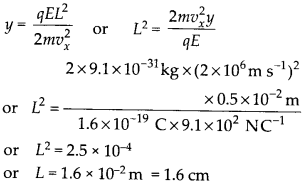All Chapter NCERT Solutions For Class12 Physics

—————————————————————————–

All Subject NCERT Solutions For Class12

*************************************************

Remark:

I think you got complete solutions for this chapter. If You have any queries regarding this chapter, please comment on the below section our subject teacher will answer you. We tried our best to give complete solutions so you got good marks in your exam.

If these solutions have helped you, you can also share Careerkundali.in to your friends.

Best of Luck!!